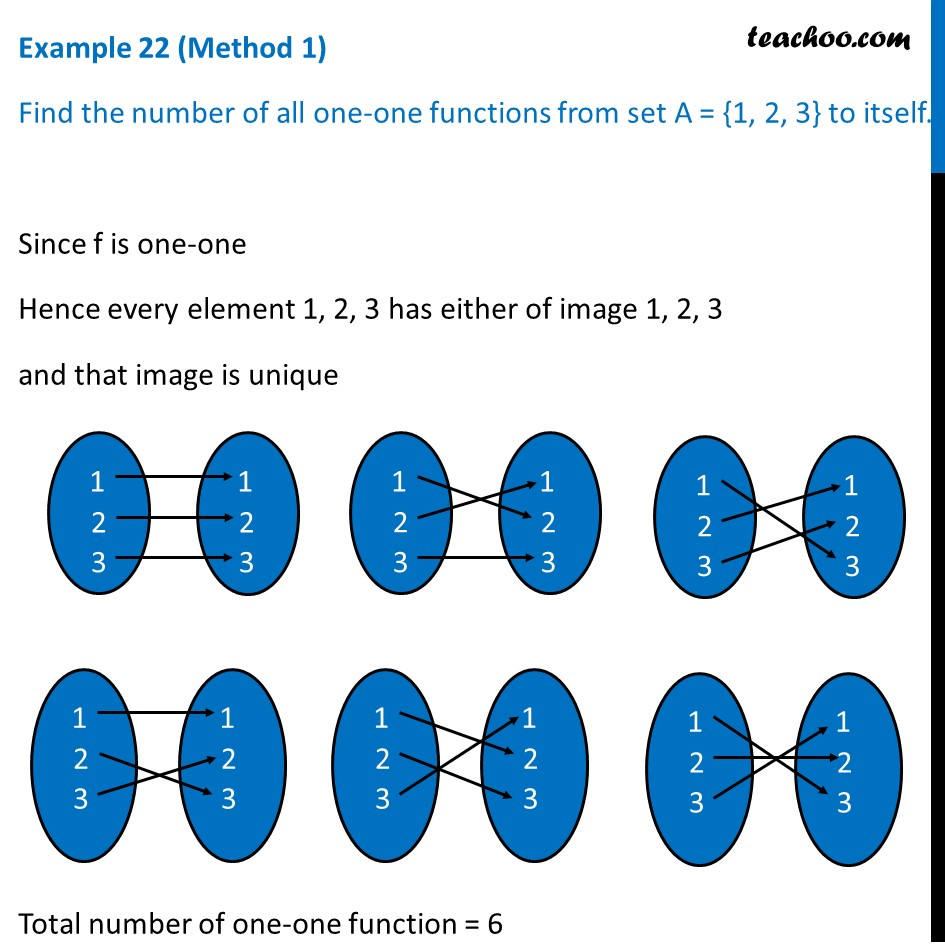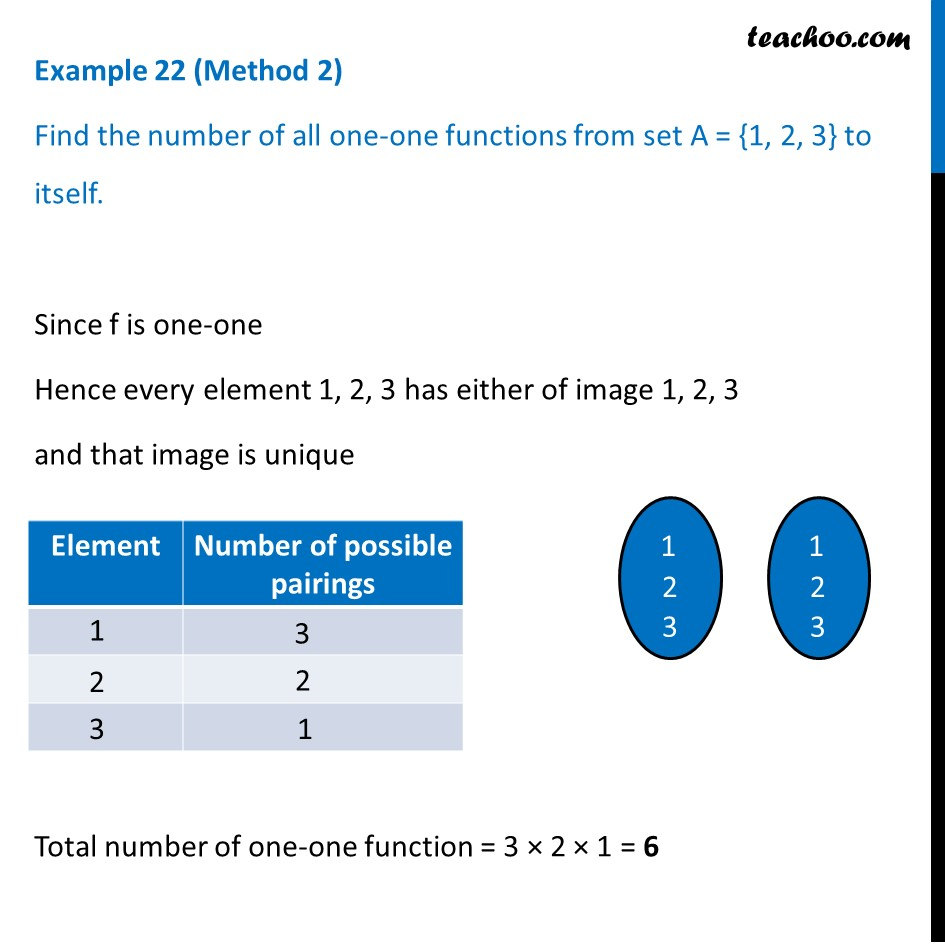To prove one-one & onto (injective, surjective, bijective)

Chapter 1 Class 12 Relation and Functions
Concept wiseLearn in your speed, with individual attention - Teachoo Maths 1-on-1 Class

### Transcript

Example 22 (Method 1) Find the number of all one-one functions from set A = {1, 2, 3} to itself. Since f is one-one Hence every element 1, 2, 3 has either of image 1, 2, 3 and that image is unique Total number of one-one function = 6 Example 22 (Method 2) Find the number of all one-one functions from set A = {1, 2, 3} to itself. Since f is one-one Hence every element 1, 2, 3 has either of image 1, 2, 3 and that image is unique Total number of one-one function = 3 × 2 × 1 = 6